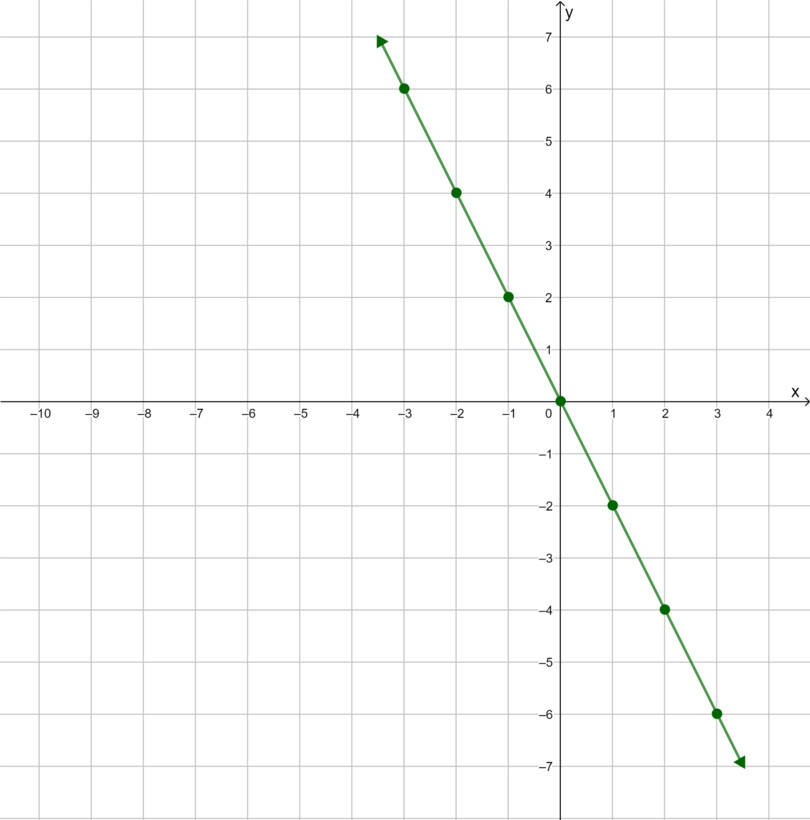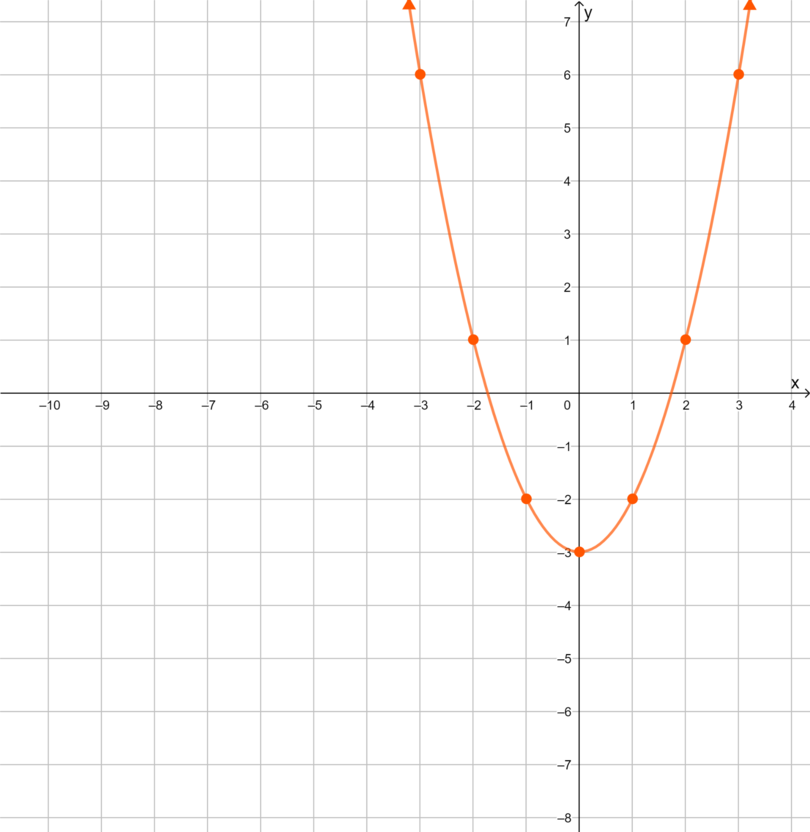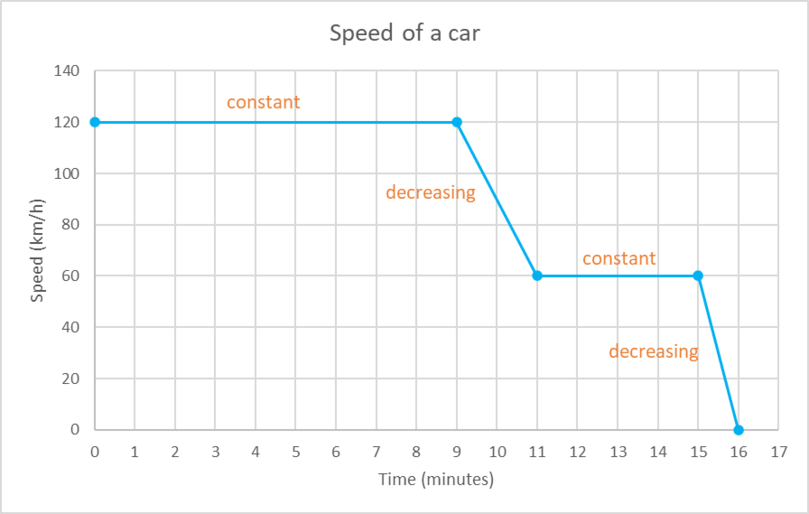Home Practice
For learners and parents For teachers and schools
Textbooks
Full catalogue
Pricing SupportLog in

We think you are located in United States. Is this correct?

# End of chapter exercises

## End of chapter exercises

 $$x$$ −3 −2 −1 0 1 2 3 $$y$$
1. Complete the table of values for the equation $$y = -2x$$.
2. Plot the ordered pairs on the Cartesian plane.
3. Draw the straight-line graph.
4. Is the graph increasing or decreasing?
1. $y = -2x$
 $$x$$ −3 −2 −1 0 1 2 3 $$y$$ 6 4 2 0 −2 −4 −6
1. and 3.2. Decreasing
 $$x$$ −3 −2 −1 0 1 2 3 $$y$$
1. Complete the table of values for the equation $$y = x^2 − 3$$.
2. Plot the ordered pairs on the Cartesian plane.
3. Draw the curve.
4. Describe some of the features of the curve.
5. Does the point $$(-5; 22)$$ lie on the curve? Use a calculation in your answer.
1. $y = x^2 − 3$
 $$x$$ −3 −2 −1 0 1 2 3 $$y$$ 6 1 −2 −3 −2 1 6
1. and 3.2. Turning point at $$(0; -3)$$. Minimum point $$(0; -3)$$. Symmetrical about the $$y$$-axis.
3. For $$x = -5$$, $$y = (-5)^2 − 3 = 25 − 3 = 22$$
Yes, the point $$(-5; 22)$$ lies on the curve.

A car travels at a constant speed of $$120 \text{ km}{\cdot}\text{h}^{-1}$$ for 9 minutes. Over the next 2 minutes, it reduces its speed at a steady rate until it reaches a speed of $$60 \text{ km}{\cdot}\text{h}^{-1}$$. The car travels at $$60 \text{ km}{\cdot}\text{h}^{-1}$$ for 4 minutes. Over the next 1 minute, it reduces its speed at a steady rate and comes to a complete stop.

1. Is the data in this situation discrete or continuous?
2. Use the information provided to draw a suitable graph.
3. On your graph indicate the sections where the graph is decreasing and constant.
1. Continuous
2. and 3.